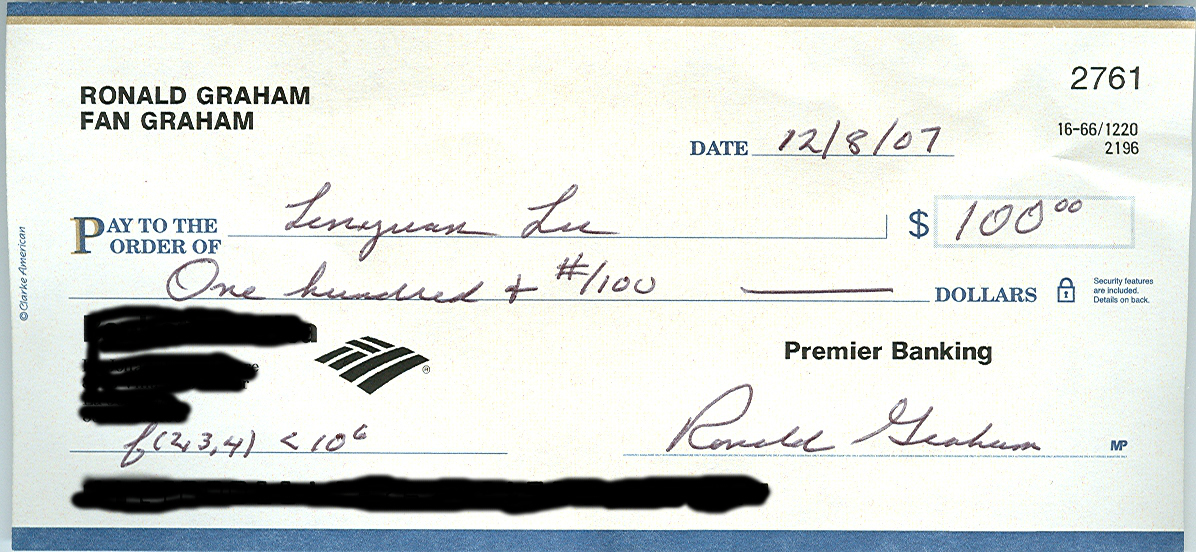## Erdos problem on Folkman graphs: f(2,3,4)

Notation: f(p,s,t) is the least integer n so that there is a simple graph G on n vertices satisfying

• G is Kt-free.
• If the edges of G is p-colored then there exists a monochromatic Ks.

History: In 1967 Erdos and Hajnal conjectured that f(p,s,t) are finite for p ≥ 2, t > s ≥ 3. The conjecture was proved (in part) for the case p=2 by Folkman in 1970, and was proved for general p by Nesetril and Rodl in 1976.
Both upper bounds of Folkman and of Nesetril-Rodl for f(2,3,4) are extremely large. Frankl and Rodl first proved f(2,3,4) < 700 billion. Erdos set a prize of \$100 for the challenge f(2,3,4) &le 10 billion. Spencer in 1988 received the prize by proving f(2,3,4)< 3 billion.

Conjecture: Erdos then conjectured f(2,3,4)<1,000,000 with a prize \$100.

Lu received the prize \$100 by proving f(2,3,4)<=9697. His paper has been published online at SIAM Journal of Discrete Math. (Vol. 21, No.4 (2008) pp. 1053-1060.)Lu received the prize from Ronald Graham on Dec. 8, 2007.Here is the check of \$100.

Recently Dudek and Rodl proved f(2,3,4)<130,000 and further improved it into f(2,3,4)<=941 and received \$50 award.